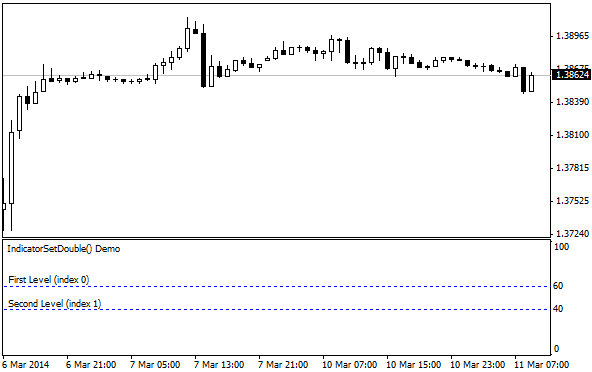﻿ IndicatorSetDouble - Custom Indicators - MQL4 Reference
English

MQL4 ReferenceCustom IndicatorsIndicatorSetDouble

# IndicatorSetDouble

The function sets the value of the corresponding indicator property. Indicator property must be of the double type. There are two variants of the function.

Call with specifying the property identifier.

 bool  IndicatorSetDouble(    int     prop_id,           // identifier    double  prop_value         // value to be set    );

Call with specifying the property identifier and modifier.

 bool  IndicatorSetDouble(    int     prop_id,           // identifier    int     prop_modifier,     // modifier    double  prop_value         // value to be set    )

Parameters

prop_id

[in]  Identifier of the indicator property. The value can be one of the values of the ENUM_CUSTOMIND_PROPERTY_DOUBLE enumeration.

prop_modifier

[in]  Modifier of the specified property. Only level properties require a modifier. Numbering of levels starts from 0. It means that in order to set property for the second level you need to specify 1 (1 less than when using compiler directive).

prop_value

[in]  Value of property.

Return Value

In case of successful execution, returns true, otherwise - false.

Note

Numbering of properties (modifiers) starts from 1 (one) when using the #property directive, while the function uses numbering from 0 (zero). In case the level number is set incorrectly, indicator display can differ from the intended one.

For example, the first level value for the indicator in a separate subwindow can be set in two ways:

• property indicator_level1  50 - the value of 1 is used for specifying the level number,
• IndicatorSetDouble(INDICATOR_LEVELVALUE, 0, 50) - 0 is used for specifying the first level.

Example: indicator that turns upside down the values ​​of levels on which the horizontal lines are placed.#property indicator_separate_window //--- set the maximum and minimum values for the indicator window #property indicator_minimum  0 #property indicator_maximum  100 //--- display two horizontal levels in a separate indicator window #property indicator_level1 25 #property indicator_level2 75 //--- set thickness of horizontal levels #property indicator_levelwidth 1 //--- set style of horizontal levels #property indicator_levelstyle STYLE_DOT //+------------------------------------------------------------------+ //| Custom indicator initialization function                         | //+------------------------------------------------------------------+ int OnInit()   { //--- two levels     IndicatorSetInteger(INDICATOR_LEVELS,2); //--- set descriptions of horizontal levels    IndicatorSetString(INDICATOR_LEVELTEXT,0,"First Level (index 0)");    IndicatorSetString(INDICATOR_LEVELTEXT,1,"Second Level (index 1)"); //--- set the short name for indicator    IndicatorSetString(INDICATOR_SHORTNAME,"IndicatorSetDouble() Demo"); //--- set clrBlue color for all levels    IndicatorSetInteger(INDICATOR_LEVELCOLOR,0,clrBlue); //---    return(INIT_SUCCEEDED);   } //+------------------------------------------------------------------+ //| Custom indicator iteration function                              | //+------------------------------------------------------------------+ int OnCalculate(const int rates_total,                 const int prev_calculated,                 const datetime &time[],                 const double &open[],                 const double &high[],                 const double &low[],                 const double &close[],                 const long &tick_volume[],                 const long &volume[],                 const int &spread[])   {    static int tick_counter=0;    static double level1=25,level2=75;    static int delta=1; //--- calculate ticks    tick_counter+=delta; //--- check ranges    if(tick_counter<0) delta=5;    if(tick_counter>25) delta=-5; //--- calculate new values    level1+=delta;    level2-=delta; //--- set new values for levels    IndicatorSetDouble(INDICATOR_LEVELVALUE,0,level1);    IndicatorSetDouble(INDICATOR_LEVELVALUE,1,level2); //--- return value of prev_calculated for next call    return(rates_total);   }HideTestIndicatorsIndicatorSetInteger